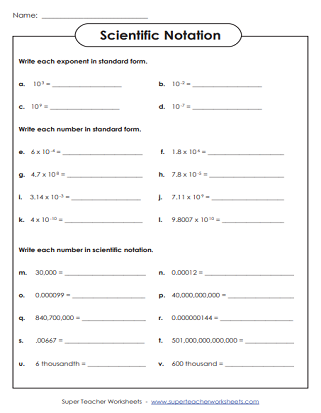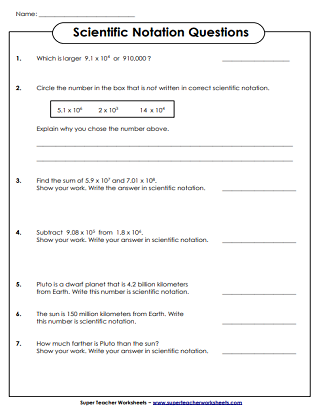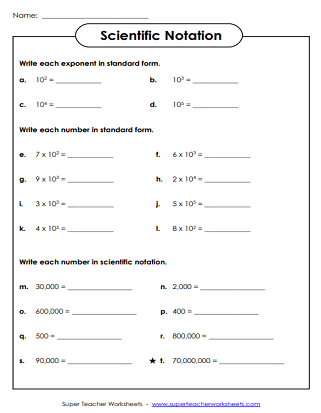# Scientific Notation

The worksheets on this page can be used to teach or review scientific notation.## Level: BasicWhole Numbers

Students convert the given numbers to and from scientific notation. This worksheet does not require students to use decimals.
Answer the math questions. Find sums, products, and differences. Most answers are be written in scientific notation.
Cut out the square-shaped number cards at the bottom of the page. Glue each number next to the equivalent in scientific notation. Basic level: whole numbers only.

## Level: IntermediateIncludes Decimals

Answer the questions. Students will compare numbers in scientific notation. They will also solve addition and subtraction problems, and write their answers in scientific notation.
Convert the given numbers to and from scientific notation. This worksheet requires students to use decimals in their scientific notation numbers.

## Level: AdvancedDecimals & Negative Exponents

Answer the questions by converting numbers to and from scientific notation.
Students convert numbers to an from scientific notation. This level includes negative exponents and decimal numbers.

Exponents

These worksheets and task cards can be used for teaching students about exponents.

Reciprocal Fractions

Practice finding the reciprocals of fractions and mixed numbers.

Math Worksheets

Super Teacher Worksheets has thousands of math worksheets, covering topics in the elementary curriculum.

## Worksheet ImagesMy Account
Site Information